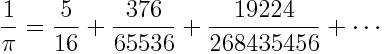# A Ramanujan series for calculating pi

Ramanujan discovered the following remarkable formula for computing π:This is not the most efficient series for computing π. My next post will give a more efficient method, also based on work of Ramanujan. But the series above is interesting for reasons explained below.

Notice that the denominator of each term in the sum above is a power of 2. This means the partial sums of the series can be represented exactly in binary. We’ll see that each term in the series adds six bits precision to the sum once we’ve added enough terms.

To understand how to use this series, we need to estimate the binomial coefficient term. Stirling’s approximation shows thatThis tells us that the nth term in the series is a rational number with numerator something like 2^6n and denominator 2^(12n+4). Therefore the nth term is on the order of 2^(-6n-4) and so the series converges quickly. The first three terms illustrates this:The error in summing up a finite number of terms is approximately the first term in the remainder, so just a few terms leads to an accurate approximation for 1/π and hence an accurate approximation for π.

To be a little more precise, when we sum the series from n = 0 to n = N, the error in approximating 1/π is on the order of the next term, 2^(-6N-10). Said another way, summing up to the Nth term computes 1/π to 6N + 10 bits. For example, suppose we wanted to compute 1/π to 200 decimal places. That’s about 664 bits, and so 108 terms of the series should be enough.

We glossed over a detail above. We said that the nth term is on the order of 2^(-6n-4). That’s true for sufficiently large n. In fact, we can say that the nth term is less than 2^(-6n-4), but only for large enough n. How large? We need the denominator term (π n)^3/2 to be larger than the numerator term 42n + 5. This happens if n is at least as large as 58.

One advantage to using this series to compute π is that you could compute later blocks of bits without having to compute the earlier blocks, provided you’re interested in the bits beyond those contributed by 58th term in the series.

Reference

Related post: Two useful asymptotic series